ISSAC 96 - Hypergeometric Function Representations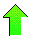Contents News View

 PFD Duplication Formula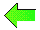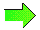The most general formula installed as a subroutine of Lookup combines the use of partial fraction decomposition and the Gamma duplication formula into a single formula.  We use the notation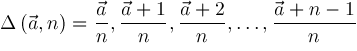in the theorem below.

Theorem Let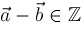,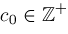,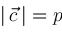,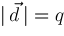,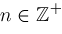,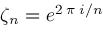, and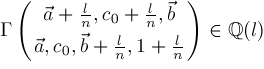have partial fraction decomposition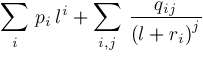Then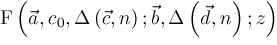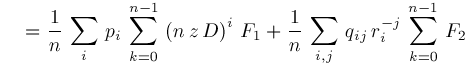where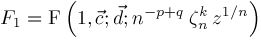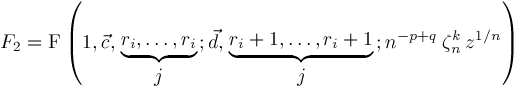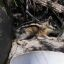©2004-2021 Planet Quantum Kelly Roach